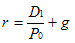## Sunday, July 13, 2008

### Relating Company Fundamentals To The Dividend Discount Model

(I originally posted the following article on The DIV-Net on July 6, 2008.)

Before I describe how to apply the Dividend Discount Model (DDM) to other fundamental company factors like, price to earnings, price to book and return on equity, following is a brief overview of the DDM.

The usefulness of valuing a stock using the Dividend Discount Model is the fact the formula provides an investor a price he/she can expect to receive from the specific stock investment. The investor receives all dividends on an ongoing basis plus the value of the stock when it is sold. In order to value the shares using the DDM, an investor calculates the present value of all the future cash flows. The constant DDM formula is:The DDM can be used to relate the value of a stock to a company's fundamentals.

Price Earnings Ratio

To get to the P/E or price to earnings ratio, both sides of the constant DDM equation need to be divided by earnings per share. The resulting formula is:

• increase the dividend payout then the P/E increases
• increase the required rate of return, r, the P/E decreases
• increase the growth rate, g, the P/E increases.
Required Rate of Return

As noted below, the DDM formula can be rearranged so an investor can ascertain that the required rate of return, r, is comprised of the dividend yield and the rate of growth.Price of a Stock

Finding the relationship between price of a stock to the book value, return on equity, required rate of return, growth rate and dividend payout ratio involves rearranging the DDM formula as follows:If B0 is the current book value and ROE is return on equity, one knows:

E0=(B0)(ROE0) since ROE0=E0/B0.

Therefore,

• book value--------------->increase B0 results in increase in P0
• ROE--------------------->increase ROE results in an increase in P0
• dividend yield-----------> increase D0/E0 results in increase in P0
• expected growth rate---->increase g results in an increase in P0
• required rate of return--->increase r results in a decrease in P0
Price to Book

Finding the relationship of fundamental company characteristics to a company's price to book ratio simply involves dividing both sides of the above equation by B0.

• increase in ROE0 increases P0/B0
• increase in D0/E0 increases P0/B0
• Increase in g increases P0/B0
• Increase in r decreases P0/B0.
In the end, using the dividend discount model provides more information than taking the future cash flows from a stock investment and discounting them back to the present via a present value calculation. Delving underneath the formula can provide insight into a company's future prospects if an investor can determine the fundamental company factors that may or may not improve in the future.

Pamela Peterson Drake, Ph.D., CFA of James Madison University has a nice site containing investment information on topics such as portfolio theory and fundamental analysis, just to name a few. Once on the site, click on the "modules" tab.

Source:

Investing in stocks (pdf)
By: Pamela Peterson Drake, Ph.D., CFA
http://peregrin.jmu.edu/~drakepp/investments/modules/module8.pdf